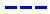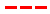Disneyland attraction wait times forecast for December 7, 2017.

•The Average Wait Time We PredictedThe Average Wait Time We Saw
•The Average Wait Time We PredictedThe Average Wait Time We Saw
•The Average Wait Time We PredictedThe Average Wait Time We Saw
•The Average Wait Time We PredictedThe Average Wait Time We Saw
•The Average Wait Time We PredictedThe Average Wait Time We Saw
•The Average Wait Time We PredictedThe Average Wait Time We Saw
•The Average Wait Time We PredictedThe Average Wait Time We Saw
•The Average Wait Time We PredictedThe Average Wait Time We Saw
•The Average Wait Time We PredictedThe Average Wait Time We Saw
•The Average Wait Time We PredictedThe Average Wait Time We Saw
•The Average Wait Time We PredictedThe Average Wait Time We Saw
•The Average Wait Time We PredictedThe Average Wait Time We Saw